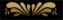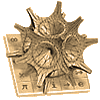### Resources:Online ToolsCourse NotesExercise SheetsComplete ReviewsMid-terms & Exams

### Must-Know's:

How much should good tutoring cost?
Review rates here

How many sessions per week is enough?
Determine it here

How long should one tutoring session be?
Find out here

### Old Websites:Design of 2007Design of 2006

##Software

Below you will find a list of various useful software that can be utilized to help solve both simple and complex mathematical problems.Statistical Discovery Software
JMP
JMP is a computer program that was developed to perform simple and complex statistical analyses. It dynamically links statistics with graphics to interactively explore, understand, and visualize data.Maple
Maplesoft
The Maple system embodies advanced technology such as symbolic computation, infinite precision numerics, innovative Web connectivity and a powerful 4GL language for solving a wide range of mathematical problems encountered in modeling and simulation.Mathematica
Wolfram Research Inc.
Mathematica is a specialized computer program used mainly in scientific and mathematical fields. It provides cross-platform support for tasks such as symbolic or numerical calculations, arbitrary precision arithmetic, data processing, and plotting.MATLAB
MathWorks
MATLAB is a numerical computing environment and programming language. It allows easy matrix manipulation, plotting of functions and data, implementation of algorithms, creation of user interfaces, and interfacing with programs in other languages.